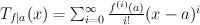## March 2010 riddle

This month's riddle is about Taylor series. The Taylor series of a function f around a point a, which we will denote Tf|a, is the following function:where f(i)(a) is the i'th derivative of f at a.

In many cases, when f is a smooth function, a Taylor series Tf|a(x) is used as a convenient approximation to f(x).

A smooth function, for our purposes, more properly termed a C function, is a function that is continuous, infinitely many times differentiable, and that all of its derivatives are also continuous, and all this in the entire domain in which it is defined.

However, even given all these requirements, it is still not guaranteed that a Taylor series converges at any particular point, x, or, if it does, that Tf|a(x)=f(x). In fact, let us define the set Χf|a to be the set of all points, x, in which Tf|a(x) exists and equals f(x).

This month's question:

f is a smooth function defined on ℜ+ (the positive real numbers). It satisfies the property that for all d ∈ ℜ+ there exist a and u ∈ ℜ+ such that Χf|a is the open segment (u,u+d).

Readers are asked to refrain from looking up any texts that give examples of Taylor series in solving this riddle. Most basic introductions to Taylor series fall into this category.

Correction: Though the riddle, as written above, has solutions, and one such solution is documented on the answer page, the original riddle was meant to be much simpler and the original solution was meant to be much more elegant. In order to allow for a simple, elegant solution, one should allow u to be nonnegative, and not only strictly positive. The restriction that u should be positive was unintended, and was put in the original wording in error.

### List of solvers:

Elegant and original solutions can be submitted to the puzzlemaster at riddlesbrand.scso.com. Names of solvers will be posted on this page. Notify if you don't want your name to be mentioned.